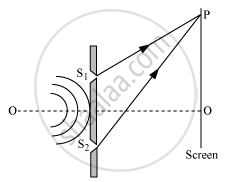# Answer the Following Question. Describe Any Two Characteristic Features Which Distinguish Between Interference and Diffraction Phenomena. Derive the Expression for the Intensity - Physics

Describe any two characteristic features which distinguish between interference and diffraction phenomena. Derive the expression for the intensity at a point of the interference pattern in Young's double-slit experiment.

#### Solution

Difference between interference and diffraction:
In the interference pattern, the intensity of the dark fringe is completely zero.
In the diffraction pattern, the intensity of secondary minima is minimum, but not completely zero.
In interference pattern the width of all the interference fringes is equal. In diffraction pattern the width of central maxima is large, and on increasing distance, the width of maxima decreases.
In interference pattern the intensity of all the bright bands is equal.
In the diffraction pattern, the intensity of all the secondary maxima is not equal.

The principle of superposition of light waves:
When two or more wave trains of light travelling in a medium superpose upon each other, the resultant displacement at any instant is equal to the vector sum of the displacements due to individual waves.

If vecy_1,vecy_2,vecy_3,.... be the displacements due to different waves, then the resultant displacement is given by, vecy = vecy_1 + vecy_2 + vecy_3 + ... conditions for constructive and destructive interference:Let  the displacement of the waves from the source S_1 and S_2 at a point P on the screen at any time 't' be given by,
"y"_1 = a_1 sin omegat
and
"y"_2 = a_2 sin(omegat + phi)
where phi is the constant phase difference between the two waves
by the superposition principal , the resultant displacement at point P is given by,

"y" = "y"_1 + "y"_2

= a_1 sin omegat + a_2 sin (omegat + phi)

= a_1 sin omegat + a_2 sin omegat cos phi + a_2 cos omegat sin phi

y = (a_1 + a_2 cos phi) sin omegat + a_2 sin phi cos omegat  ...(i)

Let a_1 + a_2 cos phi = A cos theta ...(ii)

a_2 sin phi = A sin theta  ...(iii)

Then, equation (i) becomes
"y" = Acostheta sin omegat + A sin theta cos omegat

"y" = Asin(omegat + theta)

Squaring and adding both sides of the equations (ii) and (iii), we obtain

A^2 cos^2theta + A^2 sin^2 theta = (a_1 + a_2 cos phi)^2 + a_2^2 sin^2 phi

A^2 = a_1^2 + a_2^2 (cos^2phi + sin^2phi) + 2a_1a_2 cos phi

A^2 = a_1^2 + a_2^2 + 2a_1a_2 cos phi

The intensity of light is directly proportional to the square of the amplitude of the wave. The intensity of light at point P on the screen is given by,

I = a_1^2 + a_2^2 + 2a_1a_2 cos phi     ...(iv)

Concept: Interference
Is there an error in this question or solution?